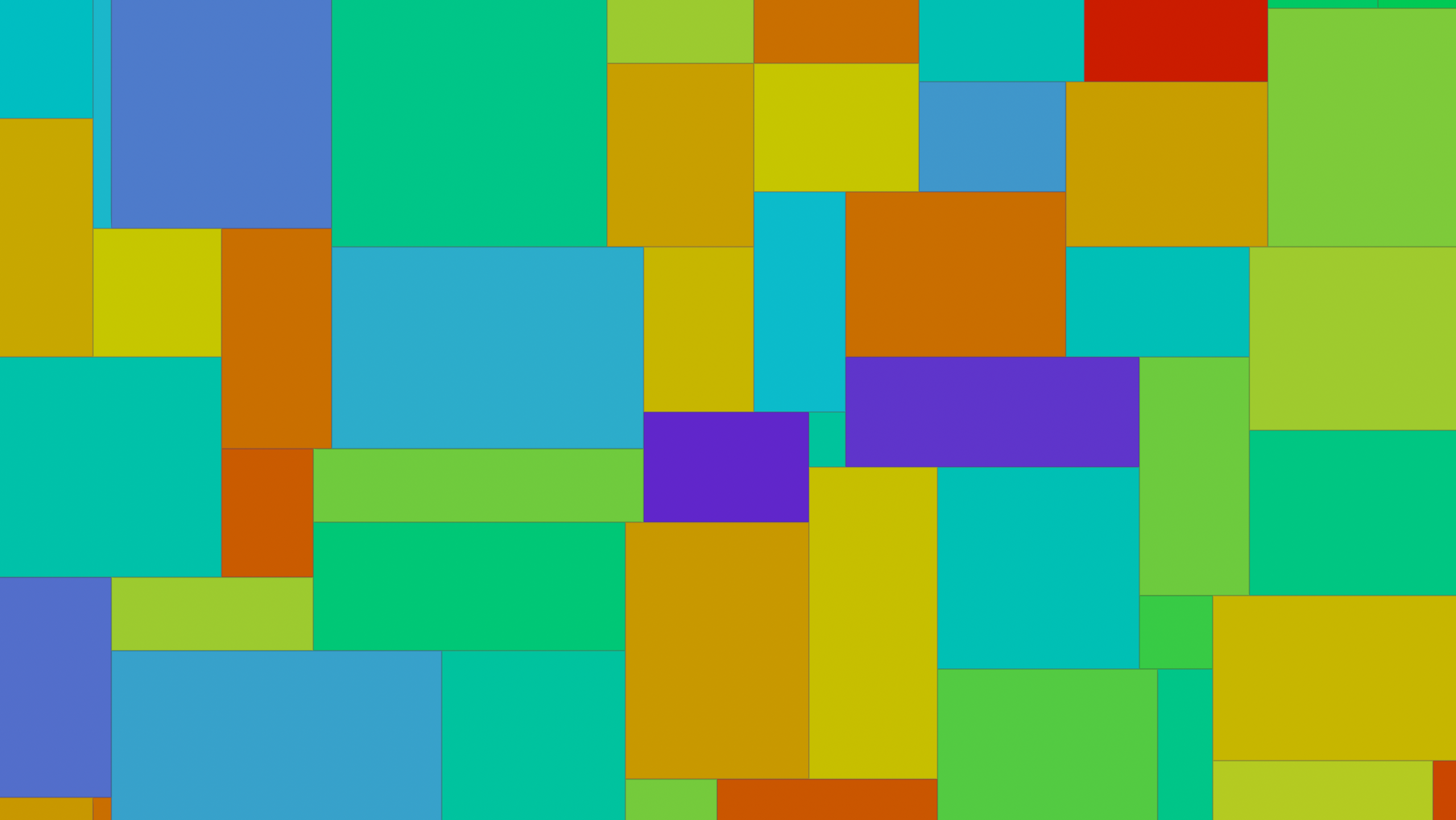# Non-overlapping Tiles

calendar_today

03.04.2022

label

Modeling, Architecture

mouse

18.0

### 1 Code

Creating non-overlapping tiles without jumping through too many loops can be quite a challenge. In such cases, code examples from the Shadertoy community can be really inspiring to us, perhaps more node based, Houdini artists.

This original code written by a user called fizzer cleverly offsets a checkerboard and supports scaling as well as shifting the entire pattern. A translation to Houdini's VEX to be used inside primitive wrangle creates tiles based on the vertex UV coordinates:

``````// PARAMETERS
float scale = 1.0 / chf('scale');
float offset_x = chf('offset_x');
float offset_y = chf('offset_y');

// UV
vector uvw = vertex(0, 'uv', i@vtxnum);
vector2 uv = (vector2(uvw) + set(offset_x, offset_y)) * scale;
vector2 f = frac(uv);
vector2 c = floor(uv);

// TILING
vector2 offset_cell;
vector2 coord_tile = c;

float r0 = rand(floor(uv));
int orientation = (int(c.x) + int(c.y)) & 1;
coord_tile[orientation] += f[orientation] > r0;
offset_cell[orientation] = (f[orientation] > r0) ? 1.0 : -1.0;

float r1 = rand(c + offset_cell);
coord_tile[orientation ^ 1] += f[orientation ^ 1] > r1 ? 1.0 : 0.0;

u@tiles = coord_tile;
v@Cd = rand(coord_tile);``````

After this, the primitives are split up by the `coord_tile` attribute and a divide node removes inner edges, which leaves us with clean tiles that can be projected onto surfaces or used as a base for texturing.